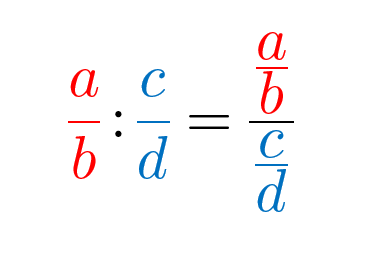# Multiplying and dividing fractions

#### How to multiply two fractions?

Simply multiply their numerators with each other and their denominators with each other.

$\displaystyle{\frac{a}{b} \cdot \frac{c}{d}} = \displaystyle{\frac{ac}{bd}}$

Example 1. Multiply the given fractions.

$\displaystyle{\frac{2}{7} \cdot \frac{3}{5} = ?}$

It doesn’t change the final result whether we first shorten the fractions and then multiply them or we first multiply them and then shorten the final result. Be careful, only the numerators can be shorten with the denominators, never numerator with numerator or denominator with denominator. But you can take any denominator and any numerator in a multiplication.

Example 2. Shorten the fractions before multiplication.

$\displaystyle{\frac{2}{7} \cdot \frac{14}{8} = ?}$
$\displaystyle{\frac{2}{7} \cdot \frac{14}{8} = \frac{2}{7} \cdot \frac{7}{4}}$

From here you can see that your numerators are $2$ and $7$, and denominators $7$ and $4$. You can shorten $7$ with $7$, and $2$ with $4$. Remember never to shorten two numerators or two denominators. From here we can easily get our solution:

$\displaystyle{\frac{2}{7} \cdot \frac{14}{8} = \frac{2}{7} \cdot \frac{7}{4} = \frac{1}{1} \cdot \frac{1}{2} = \frac{1}{2}}$.

#### How to divide two fractions?

The easiest way to divide two fractions is to use a reciprocal value of the divisor to switch from divison to multiplication.

$\displaystyle{\frac{a}{b} : \frac{c}{d} = \frac{a}{b} \cdot \frac{d}{c}}$

Remember that division is not a commutative operation, which means that you have to be careful of which reciprocal value you take- always from the divisor.

Example 3. Divide two fractions.

$\displaystyle{\frac{1}{2} : \frac{5}{8} = \frac{1}{2} \cdot \frac{8}{5} = \frac{4}{5}}$

There is another way to divide fractions, to make a complex fraction. A complex fraction has fractions as numerators and denominators, and while writing them you have to be careful and in every point know which fraction line is your main fraction line.

As you know, every division can be written as a fraction $\displaystyle{\frac{a}{b} = a : b}$.So how do you solve this? You multiply by the rule – external with external and internal with internal, that is the numerator of the first fraction with the denominator of the second, and the denominator of the first with the numerator of the second fraction. The numerator of the quotient will be the product of external numbers, and denominator the product of internal numbers.Example 4. Divide two fractions using complex fraction notation.

$\displaystyle{\frac{(1)}{2} : \frac{5}{4} = ?}$
$\displaystyle{\frac{1}{2} : \frac{5}{4} = \frac {\frac{1}{2}}{\frac{5}{4}} = \frac{(1 \cdot 4)}{(2 \cdot 5)} = \frac{2}{5}}$